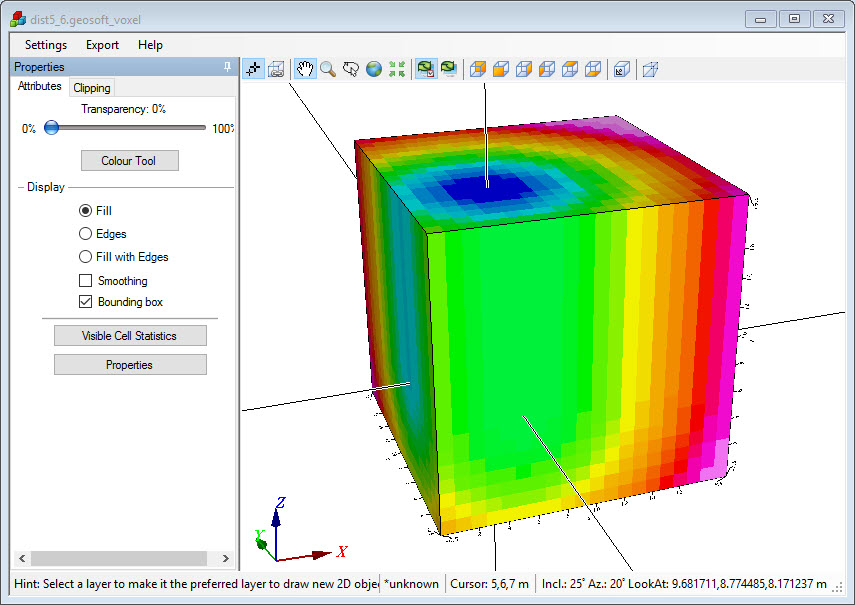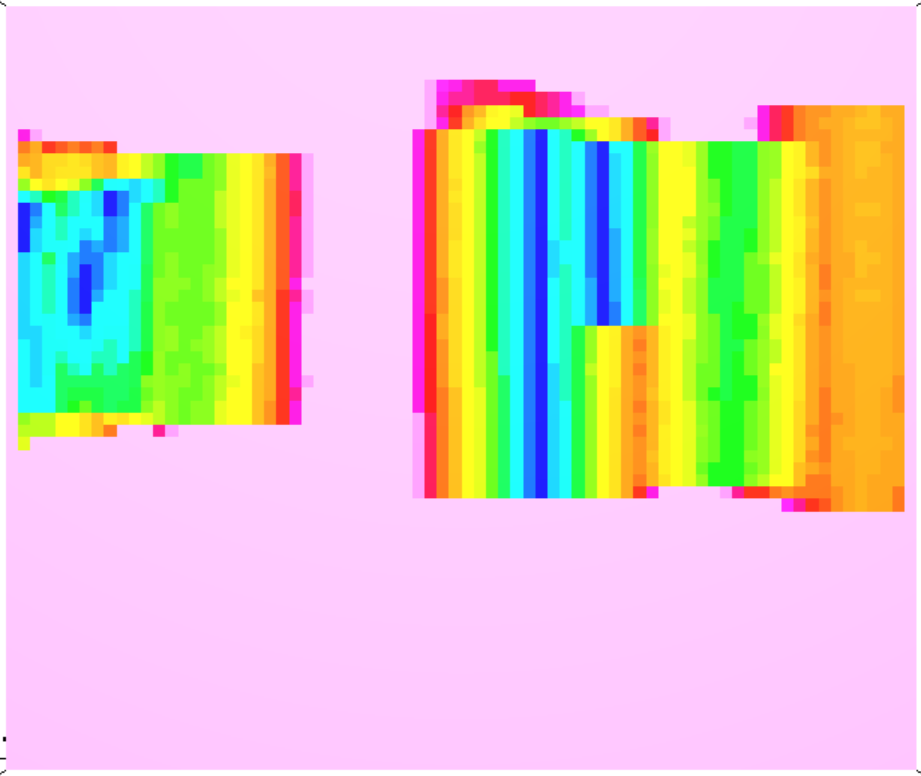# Compute voxel of distances to drill holes

Is there anyway to generate a 3D voxel model with cells values that show the distance to the nearest drill hole, even if it requires a couple of steps?
Tagged:

• Hi @NickWilliams, I'm trying to think this through and find a relatively simple workaround for you, but nothing has really worked out yet. What are you planning to use this for? A VOXI constraint?

Here's one idea I haven't worked all the bugs out of yet but I think is doable:

Use voxel direct gridding and blanking distance to create a series of voxels that have increasing blanking distance as you get farther from the drillhole. The first voxel would be directly over the drillhole traces. The 2nd would be 1 cell away, the 3rd voxel would be 2 cells away, etc.

Then use voxel math to nestle them one inside the other. It would be sort of chunky and maybe not as smooth a transition of distance that you were looking for. Is this what you were looking for?

Does that explanation make sense? Should I continue down this path? What sort of distances are you interested in? How far from the drillhole are you interested in seeing for example? And are you working in an area with a big cluster of drillholes, and what's the average distance between them?

Community Manager• Assuming a vertical drill hole, you can use a voxel math expression to compute the distance for a single drill hole:
V0 = (z>=z_bottom)?(sqrt((x-X0)**2 + (y-Y0)**2)):(sqrt((x-X0)**2 + (y-Y0)**2+ (z-z_bottom)**2));
Where X0, Y0 is the location of the drill hole and z_bottom is the bottom.

You can merge the results from individual drill holes by using voxel math to take the minimum distance value between the two.

Here is an image created by using the expression above with X0=5, Y0=6, and z_bottom=7.Senior Scientist - Potential Fields• @NickWilliams , If your drill hole path is curving and you have a VOXI subscription here is another approach.
• Create a VOXI document for the desired Voxel coverage (you wont be running an inversion).
• Inside the VOXI document run Constraints>Create>Drillhole Weight Models.
• Create a drillhole constraint using a GDB that has the XYZ Path of the drill hole and some property data channel. The data is not really important. The GDB and AoI must have the same projection and of course coincide.
• Expand the More of the Constraint dialog and give it a very large "Radius around drillholes".
• Keep the default values of the other parameters.
• 2 voxels will be produced, Ignore the "Parameter Weight model". You want the "Weight model" .
• The values of the weight voxel for the cells that the drillhole crosses is ~0.9 and decrease as a function of distance exponentially away from the drillhole in the plane normal to the drill hole trace . The weight values are calculated using the equation below. From this equation you can figure out the voxel math equation to produce the distance-from-drillhole voxel .Technical Product Manager, Geophysical• Thanks all. I will typically be using many curved drill holes. I'll try these out. The main purpose at this stage is for visualization (ie, show me anomalies >100 m from a drill hole), but if I can be done easily there are also some interesting options for applying different sorts of constraints to VOXI models.
• @ElizabethBaranyi I tested this approach and it works well. A few notes though:
1. The parameter 'd' in the formula you posted above is measured in "cells". It must be multiplied by the cell size to get the actual distance.
2. The calculation only works to a distance of 10 cells from any drill hole.
3. It looks like the calculation only takes into account the lateral distance to a drill hole on any layer of the mesh instead of using true 3D distances. It produces cylinders with flat tops and flat bottoms instead of producing a hemisphere at the end of each drill hole.It's a very good start and will serve most purposes.